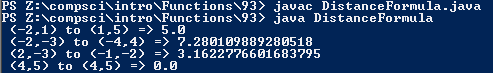# Assignment #93 and DistanceFormula

## Code

```      /// Name: Jason Kim
/// Period: 7
/// Program Name: DistanceFormula
/// File Name: DistanceFormula.java
/// Date Finished:12/14/15

public class DistanceFormula
{
public static void main( String[] args )
{
double d1 = distance(-2,1 , 1,5);
System.out.println(" (-2,1) to (1,5) => " + d1 );

double d2 = distance(-2,-3 , -4,4);
System.out.println(" (-2,-3) to (-4,4) => " + d2 );

System.out.println(" (2,-3) to (-1,-2) => " + distance(2,-3,-1,-2) );

System.out.println(" (4,5) to (4,5) => " + distance(4,5,4,5) );
}

public static double distance( int x1, int y1, int x2, int y2 )
{
double D;
D = Math.sqrt((x1-x2)*(x1-x2) + (y1-y2)*(y1-y2));
return D;
}
}

```

### Picture of the output